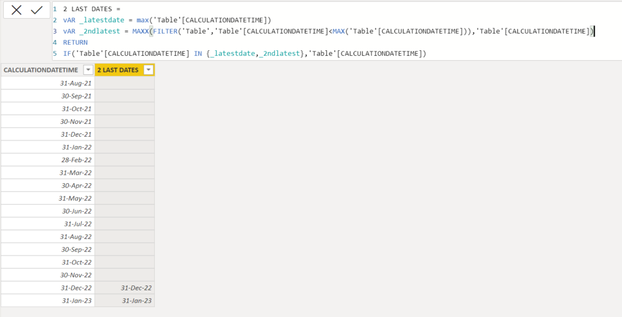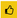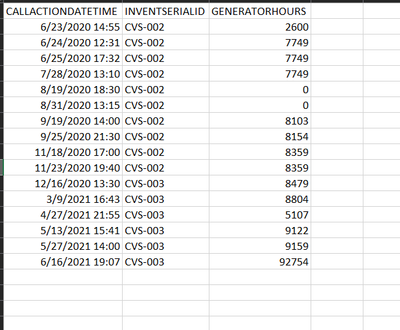cancel
Showing results for
Did you mean:Helper II

## how to get the latest date and 2nd latest date in Power BIIf I have the above table I would want to get just the latest date : 6/16/2021 and second latest date : 5/27/2021.

can you please provide me solution.

13 REPLIES 13Super User

Try these measures:

latest date = max('Table'[CALCULATIONDATETIME])

2nd latest = MAXX(FILTER('Table','Table'[CALCULATIONDATETIME]<MAX('Table'[CALCULATIONDATETIME])),'Table'[CALCULATIONDATETIME])

Example:Appreciate your Kudos!!Helper II

Hi Vahid,

I want them in the same column, just sort the last and secondlast date from calculationdatetime column. so basically column should have only 2 dates.Super User

``````2 LAST DATES =
vAR _latestdate = max('Table'[CALCULATIONDATETIME])
vAR _2ndlatest = MAXX(FILTER('Table','Table'[CALCULATIONDATETIME]<MAX('Table'[CALCULATIONDATETIME])),'Table'[CALCULATIONDATETIME])
RETURN
IF('Table'[CALCULATIONDATETIME] IN {_latestdate,_2ndlatest},'Table'[CALCULATIONDATETIME])``````

the output will be as below:Appreciate your Kudos!!Helper II

Sorry for the confusion, I would be needing the last 2 dates for each inventserialid, so table shows just CVS-002, but there are other ids as well, so for each of the ids I would be requiring last 2 dates.Super User

Try this measure:

``````2 LAST DATES =
Var _invent = FIRSTNONBLANK('Table'[INVENTSERIALID],"")
vAR _latestdate = CALCULATE(max('Table'[CALCULATIONDATETIME]),ALLEXCEPT('Table','Table'[INVENTSERIALID]))
vAR _2ndlatest = MAXX(FILTER(filter('Table','Table'[INVENTSERIALID]=_invent),'Table'[CALCULATIONDATETIME]<_latestdate),'Table'[CALCULATIONDATETIME])
RETURN
IF('Table'[CALCULATIONDATETIME] IN {_latestdate,_2ndlatest},'Table'[CALCULATIONDATETIME])``````

the output will be as below:Appreciate your Kudos!!Super User

Can you pleae share the sample file here.

Table with different "inventserialid" or Power BI file.Helper IIHere is the tableSuper User

Try this measure:

``````2 LAST DATES =
Var _invent = FIRSTNONBLANK('Table'[INVENTSERIALID],"")
vAR _latestdate = CALCULATE(max('Table'[CALCULATIONDATETIME]),ALLEXCEPT('Table','Table'[INVENTSERIALID]))
vAR _2ndlatest = MAXX(FILTER(filter('Table','Table'[INVENTSERIALID]=_invent),'Table'[CALCULATIONDATETIME]<_latestdate),'Table'[CALCULATIONDATETIME])
RETURN
IF('Table'[CALCULATIONDATETIME] IN {_latestdate,_2ndlatest},'Table'[CALCULATIONDATETIME])``````

the output will be as below:Appreciate your Kudos!!Helper II

Hi,

i got this error when i tried to create the column

"DAX comparison operations do not support comparing values of type Text with values of type Date. Consider using the VALUE or FORMAT function to convert one of the values."Super User

It works well on my computer! Make sure the "CALCULATIONDATETIME" column format is Date.

Is it possible to share your file with me?

Appreciate your Kudos!!Helper II

Since im new to forum im unable to upload power bi file here. So the inventserialid is text, seems like that is causing problem.  CALCULATIONDATETIME is in date format alreadySuper User

It seems everything is OK!Helper II

I tried the formula and it worked, but for few it shows blank or doesnt pick up the 2nd latest date.